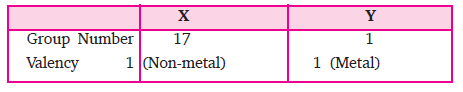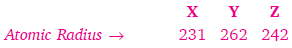# Important Questions For Class 10 Science Chapter 5- Periodic Classification of Elements

Q1. Give one example of Döbereiner’s Triad.

Ans.   Li,        Na,           K

7          23           39

Q2. How many triads could Döbereiner identify from the existing elements then?

Ans. Döbernier could identify only three triads.

Q3. What is the limitation of Döbereiner triads?

Ans. He failed to arrange all the elements in triads having same chemical properties.

Q4. What was the basis of classification of elements made by Newlands?

Ans. Newlands arranged the elements in the order of increasing atomic masses.

Q5. Give two limitations of Newlands’ law of Octaves.

Ans. Two limitations of Newlands’ law of octaves are:

(i) The law was applicable only upto calcium.

(ii) In order to fit elements into his table, Newlands adjusted two elements in wrong slot, and grouped unlike elements in same groups.

Q6. On what basis did Mendeleev classified the element?

Ans. Mendeleev arranged the elements on the basis of their increasing atomic mass and similarity of chemical properties.

Q7. Which two chemical properties were considered by Mendeleev for grouping of elements?

Ans. The two chemical properties are:

(a) The nature of compounds formed by elements with oxygen.

(b) The nature of compounds formed by elements with hydrogen.

Q8. State Mendeleev’s Periodic Law.

Ans. The properties of elements are the Periodic Function of their atomic masses.

Q9. Define ‘groups and periods’.

Ans. The vertical columns in a Periodic Table are called groups and the horizontal rows are called periods.

Q10. What is the formula of oxide and hydride of Group I elements?

Ans. Oxide formula → R2O

Hydride formula → RH.

‘R’ represents element.

Q11. Name three elements discovered later, which filled gaps left by Mendeleev for them.

Ans. Scandium, gallium and germanium.

Q12. What are isotopes?

Ans. Isotopes are the atoms of same element having same atomic number but different mass number.Q13. How many groups and periods are present in the Modern Periodic Table?

Ans. Modern Periodic Table has 18 groups and 7 periods.

Q14. What is the location of metals and non-metals in the Modern Periodic Table?

Ans. Metals are placed on the left side and non-metals are placed on the right side of the Periodic Table.Q15. State Modern Periodic Law.

Ans. ‘Properties of elements are a periodic function of their atomic number’.

Q16. In Modern Periodic Table what is common among all the elements in a group.

Ans. All elements in same group shows same valence electrons and same chemical properties.

Q17. Fluorine (F) atomic number 9 and chlorine (Cl) atomic number = 17 are placed in group number17, what are the number of valence electrons present in them.

Ans. Fluorine atomic number 9 = 2, 7

Chlorine atomic number 17 = 2, 8, 7

Both of them show 7 valence electron.

Q18. What is common among all the elements present in one period?

Ans. All the elements in same period show same number of shells e.g., all elements in period 3, show 3 electron shells each.

Q19. How many elements are present in first, second, third and fourth period?Q20. What is atomic size?

Ans. The radius of an atom, i.e., the distance between the centre of the nucleus and the outermost shell of an atom is called atomic size.

The atomic radius is measured in picometre. (1 pm = 10–12 m)

Q21. What happens to the size of atom as we move from left to right in a period.

Ans. The atomic size in a period decreases as we move from left to right.

Q22. How does the tendency to lose electrons will change in a period.

Ans. The tendency to lose electrons will decrease across a period as the effective nuclear charge acting on the valence shell electrons increases.

Q23. How do you think the tendency to lose electrons will change in a group?

Ans. Down the group, the effective nuclear charge experienced by valence electrons decreases, hence they can easily lose electrons.

Q24. How was the anomaly in arrangement of elements in the Mendeleev’s Periodic Table removed?

Ans. When elements were arranged in the increasing order of atomic number. The anomalies of Mendeleev’s Periodic Table were removed.

Q25. What are noble gases/inert gases?

Ans. The element which is inactive, does not react with any other element and it has its outermost shell completely filled are called inert gases or noble gases.

e.g., He, Ne, Ar, Xe.

Q26. Name two alkali metals present in Group I.

Ans. Alkali metals are Li, Na, K.

Q27. An element ‘X’ belongs to II group and 2nd period. Write the atomic number and name of element.

Ans. K L ∴ Atomic Number = 4

2, 2 Element = Beryllium

Q28. An element ‘A’ has atomic number 11, name the period and group number to which it belongs.

Q29. An element ‘P’ belongs to group = 2 and period = 3, state whether it is a metal or nonmetal and nature of its oxides.

Ans. Group 2 = Metals

Nature of oxide = Basic oxide

Q30. The electronic configuration of an atom is 2, 8, 7. Give its atomic number, nature of oxide.

Ans. Electronic configuration = 2, 8, 7

∴ Atomic number = 17

Nature of oxide = Acidic oxide

Q31. An element belongs to group 13 and period 3, name the element and give its valency.

Ans. The element is Aluminium.

The valency = 3

Q32. What are metalloids? Give 2 examples.

Ans. The elements which show some properties of metal and some properties of non-metal are called semi-metals or metalloids.

Example — Boron, Silicon, Germanium, Arsenic.

Q33. An element X belongs to group 17 and element Y belongs to group 1. What type of bond will they form?

Ans.Both of them will form ionic bond with the formula XY.

Q34. The following elements belong to same period arrange them in order.Ans.               Y              Z               X

262          242           231

The atomic size decreases in a period

Q35. What is the valency of magnesium with atomic number 12 and nitrogen with atomic number 7?

Ans. Magnesium, atomic number = 12

Electronic configuration = 2, 8, 2

∴ Valency = 2

Nitrogen, atomic number = 7

Electronic configuration = 2, 5

∴ Valency = 3

Q36. How many shells are present in all the elements that belong to period 3?

Ans. All elements in period 3 contain 3 shells in which the electrons are distributed (K, L, M).

Q37. What happens to the electropositive character of elements as we move from left to right of the period in the Periodic Table?

Ans. On moving from left to right in a period, the electropositive character decreases as the tendency to lose electrons decreases.

Q38. Fluorine, chlorine, bromine belong to same group. What is common between them?

Ans. All three elements i.e. fluorine, chlorine, bromine, have same number of valence electrons and same valency.

Q39. What are halogens? Where are they located in the Periodic Table?

Ans. Halogens are those elements which react with metals to form salts. They are present in 17th group of the Periodic Table.

Q40. Atomic number of 4 elements is given below which element will belong to the group of Helium.

W         X           Y            Z

8         15         36          20

Ans. Element Y, with atomic number 36 will belong to the same group as He.

Both are inert gas.

atomic number of Y 36 = 2, 8, 18, 8

atomic number of helium 4 = 2, 2

Announcements

Join our Online JEE Test Series for 499/- Only (Web + App) for 1 Year

Join our Online NEET Test Series for 499/- Only for 1 Year